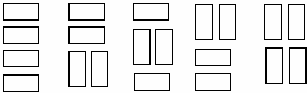# Tiling a Grid With Dominoes

Time Limit: 1000MS

Memory Limit: 65536K

## Description

We wish to tile a grid 4 units high and N units long with rectangles (dominoes) 2 units by one unit (in either orientation). For example, the figure shows the five different ways that a grid 4 units high and 2 units wide may be tiled.Write a program that takes as input the width, W, of the grid and outputs the number of different ways to tile a 4-by-W grid.

## Input

The first line of input contains a single integer N, (1 ≤ N ≤ 1000) which is the number of datasets that follow. Each dataset contains a single decimal integer, the width, W, of the grid for this problem instance.

## Output

For each problem instance, there is one line of output: The problem instance number as a decimal integer (start counting at one), a single space and the number of tilings of a 4-by-W grid. The values of W will be chosen so the count will fit in a 32-bit integer.

## Sample Input

3
2
3
7

## Sample Output

1 5
2 11
3 781

## Source

Greater New York Regional 2007